{{announcement.body}}
{{announcement.title}}

# Anaconda Python Tutorial: Everything You Need to Know

DZone 's Guide to

# Anaconda Python Tutorial: Everything You Need to Know

### Get started with this powerful data analysis platform.

· Big Data Zone ·
Free Resource

Comment (1)

Save
{{ articles.views | formatCount}} ViewsAnaconda is a data science platform for data scientists, IT professionals, and business leaders. It is a distribution of Python, R, etc. With more than 300 packages for data science, it quickly became one of the best platforms for any project. In this tutorial, we will discuss how we can use Anaconda for Python programming. The following are the topics discussed in this blog:

• Introduction To Anaconda.
• Installation And Setup.
• How To Install Python Libraries In Anaconda?
• Anaconda Navigator.
• Use cases:
You may also like: Making Python on Apache Hadoop Easier with Anaconda and CDH.

## Introduction To Anaconda

Anaconda is an open source distribution for Python and R. It is used for data science, machine learning, deep learning, etc. With the availability of more than 300 libraries for data science, it becomes fairly optimal for any programmer to work on Anaconda for data science.Anaconda helps in simplified package management and deployment. Anaconda comes with a wide variety of tools to easily collect data from various sources using various machine learning and AI algorithms. It helps in getting an easily manageable environment setup which can deploy any project with the click of a single button.

Now that we know what Anaconda is, let’s try to understand how we can install it and set up an environment to work on our systems.

## Installation And Setup

To install Anaconda, you can go here.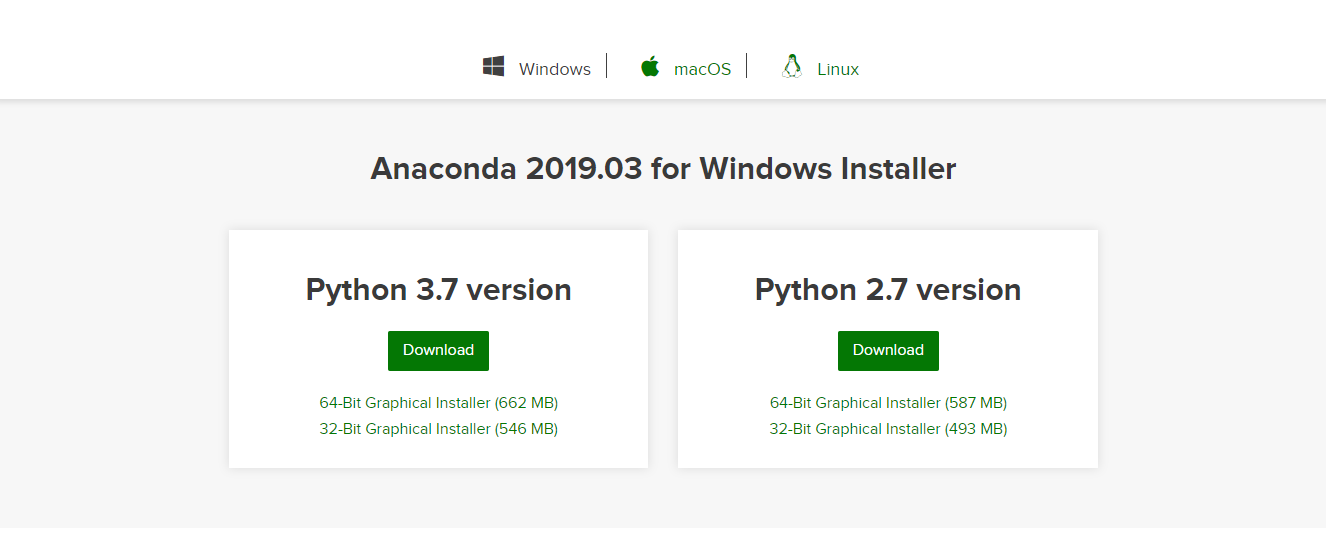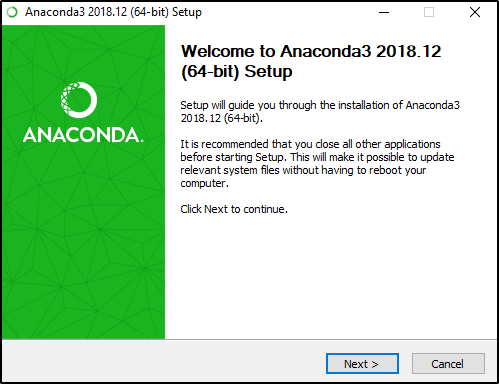Follow the instructions in the setup. Don’t forget to click on add Anaconda to your path environment variable. After the installation is complete, you will get a window like the one shown in the image below.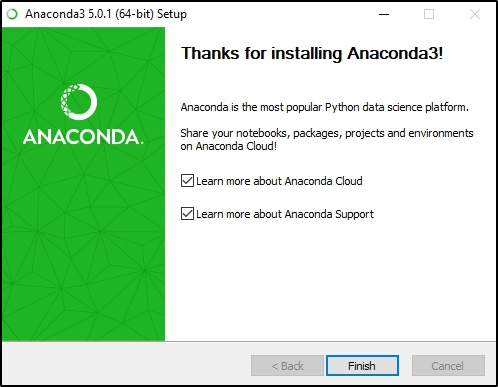After finishing the installation, open the Anaconda prompt and type `jupyter notebook`.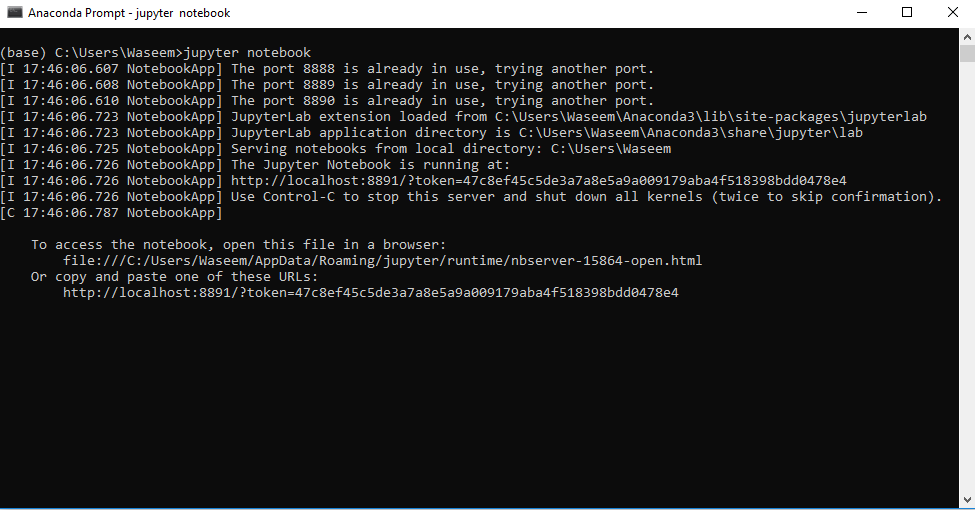You will see a window like shown in the image below.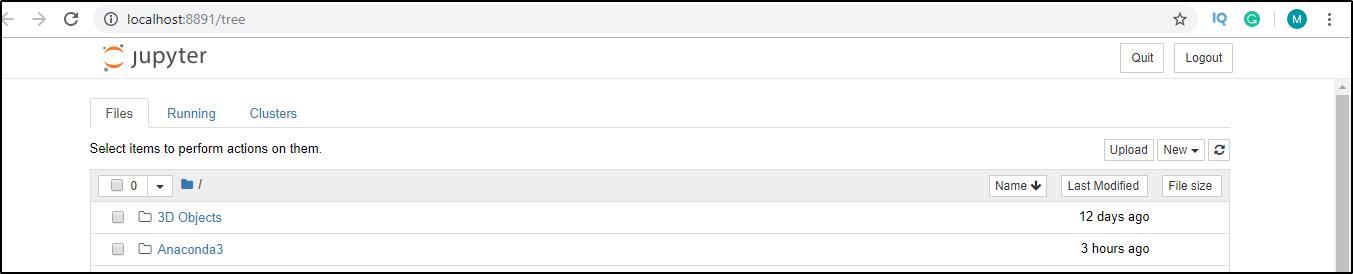Now that we know how to use anaconda for python lets take a look at how we can install various libraries in anaconda for any project.

## Install Python Libraries in Anaconda

Open the Anaconda prompt and check if the library is already installed or not.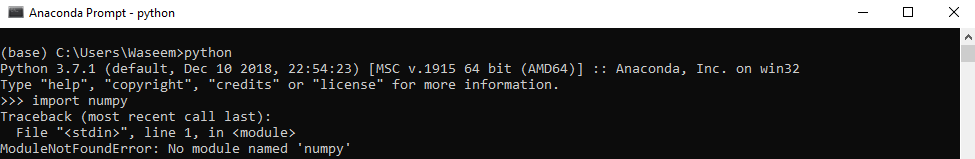Since there is no module named `numpy` present, we will run the following command to install `numpy`.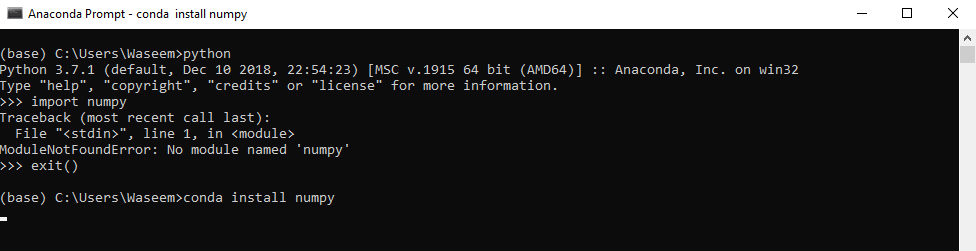You will get the window shown in the image once you complete the installation.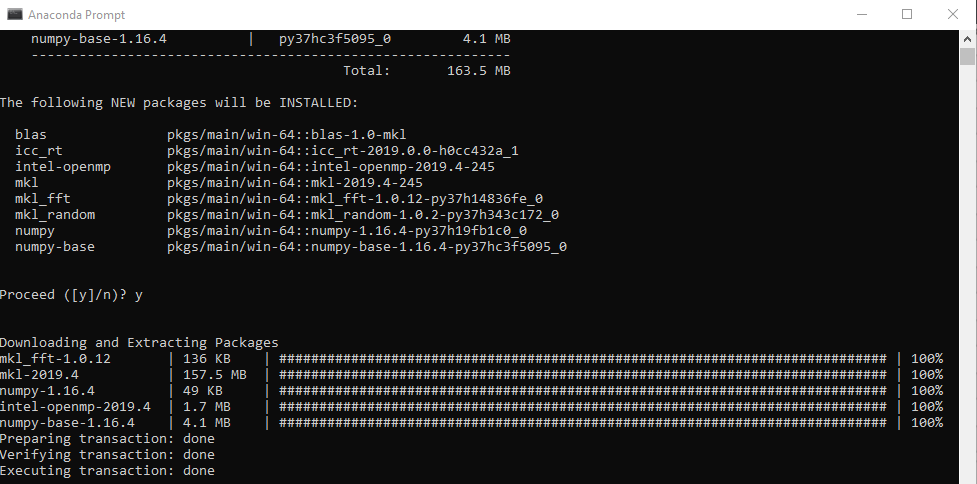Once you have installed a library, just try to import the module again for assurance.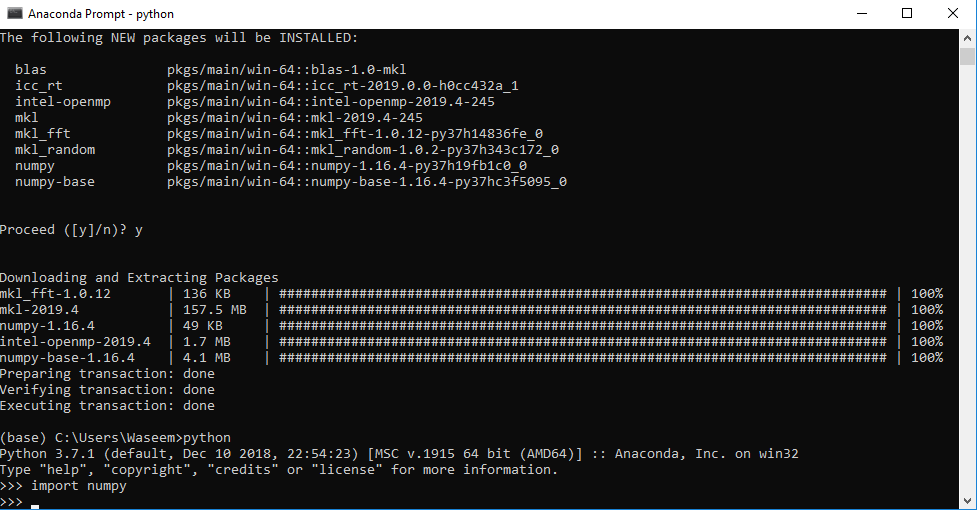As you can see, there is no error that we got in the beginning, so this is how we can install various libraries in Anaconda.

## Anaconda Navigator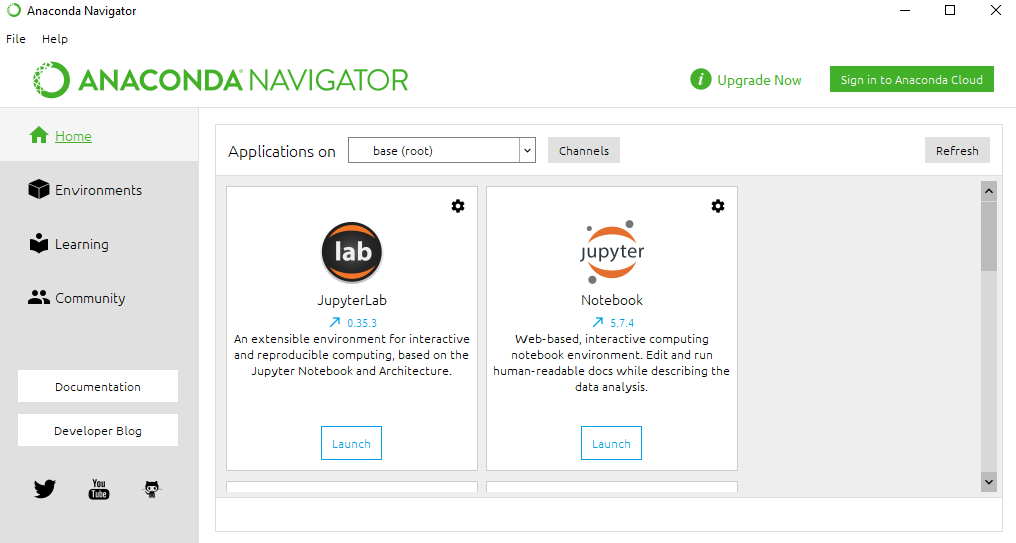Anaconda Navigator is a desktop GUI that comes with the Anaconda distribution. It allows us to launch applications and manage conda packages and environments without using the command-line.

## Python Fundamentals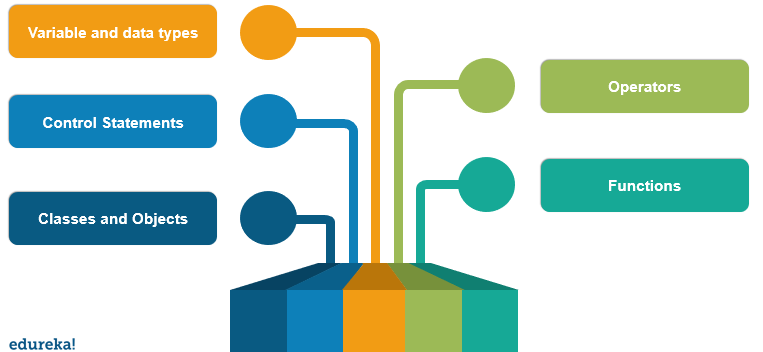### Variables and Data Types

Variables and data types are the building blocks of any programming language. Python has six data types depending upon the properties they possess. List, dictionary, set, and tuple are the collection data types in Python.

The following is an example of how variables and data types are used in Python.

``````#variable declaration
name = "Edureka"
f = 1991
print("python was founded in"  , f)
#data types
a = [1,2,3,4,5,6,7]
b = {1 : 'edureka' , 2: 'python'}
c = (1,2,3,4,5)
d = {1,2,3,4,5}
print("the list is" , a)
print("the dictionary is" , b)
print("the tuple is" , c)
print("the set is " , d)``````

### Operators

Operators in Python are used for operations between values or variables. There are seven types of operators in Python.

• Assignment Operator.
• Arithmetic Operator.
• Logical Operator.
• Comparison Operator.
• Bit-wise Operator.
• Membership Operator.
• Identity Operator.

The following is an example of the use of a few operators in Python.

``````a = 10
b = 15
#arithmetic operator
print(a + b)
print(a - b)
print(a * b)
#assignment operator
a += 10
print(a)
#comparison operator
#a != 10
#b == a
#logical operator
a > b and a > 10
#this will return true if both the statements are true. ``````

### Control Statements

Statements like `if``else``break`, and `continue` are used as control statements to gain control over the execution for optimal results. We can use these statements in loops in Python for controlling the outcome. The following is an example to show how we can work with control and conditional statements.

``````name = 'edureka'
for i in name:
if i == 'a':
break
else:
print(i)``````

### Functions

Python functions provide code reusability in an efficient way, where we can write the logic for a problem statement and run a few arguments to get the optimal solutions. The following is an example of how we can use functions in python.

``````def func(a):
return a ** a
res = func(10)
print(res)``````

### Classes And Objects

Since Python supports object-oriented programming, we can work with classes and objects as well. The following is an example of how we can work with classes and objects in python.

``````class Parent:
def func(self):
print('this is parent')

class Child(Parent):
def func1(self):
print('this is child')

ob = new Child()
ob.func()``````

These are a few fundamental concepts in Python to start with. Now, talking about the larger package support in Anaconda, we can work with a lot of libraries. Let’s take a look at how we can use python anaconda for data analytics.

## Analytics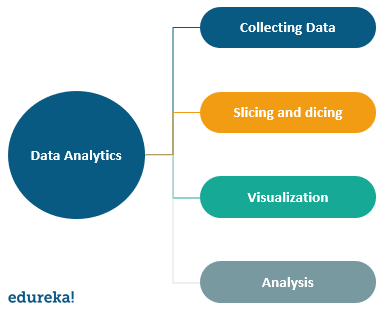These are certain steps involved in data analysis. Let’s take a look at how data analysis works in anaconda and various libraries that we can use.

### Collecting Data

The collection of data is as simple as loading a CSV file in the program. Then we can make use of the relevant data to analyze particular instances or entries in the data. The following is the code to load the CSV data in the program.

``````import pandas as pd
import numpy as np
import matplotlib.pyplot as plt
import seaborn as sns

### Slicing and Dicing

After we load the data set in the program, we must filter the data with a few changes — eliminating null values and unnecessary fields that may cause ambiguity in the analysis.

The following is an example of how we can filter the data according to the requirements.

``````print(df.isnull().sum())
#this will give the sum of all the null values in the dataset.
df1 = df.dropna(axis=0 , how= 'any')
#this will drop rows with null values``````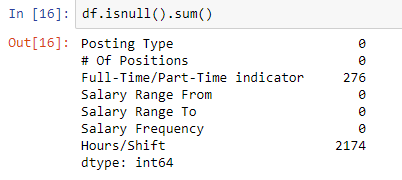We can drop the null values as well.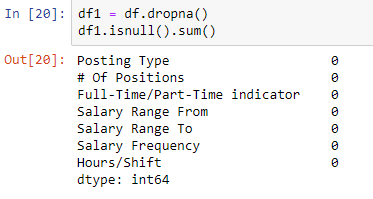#### Box Plot

``````sns.boxplot(x=df['Salary Range From'])
sns.boxplot(x=df['Salary Range To'])
``````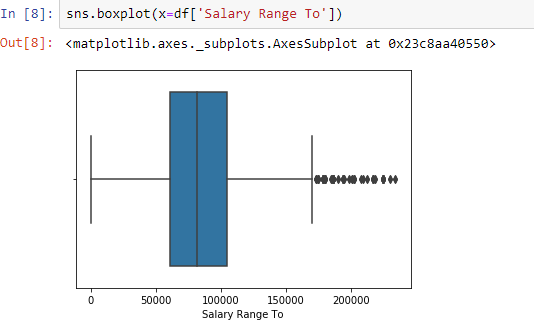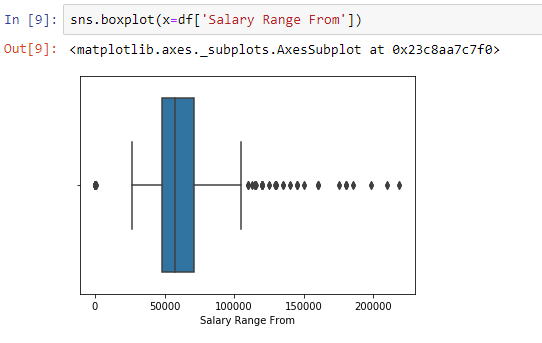#### Scatter Plot

``````import matplotlib.pyplot as plt
fig, ax = plt.subplots(figsize=(16,8))
ax.scatter(df['Salary Range From'] , df['Salary Range To'])
ax.set_xlabel('Salary Range From')
ax.set_ylabel('Salary Range TO')
plt.show()``````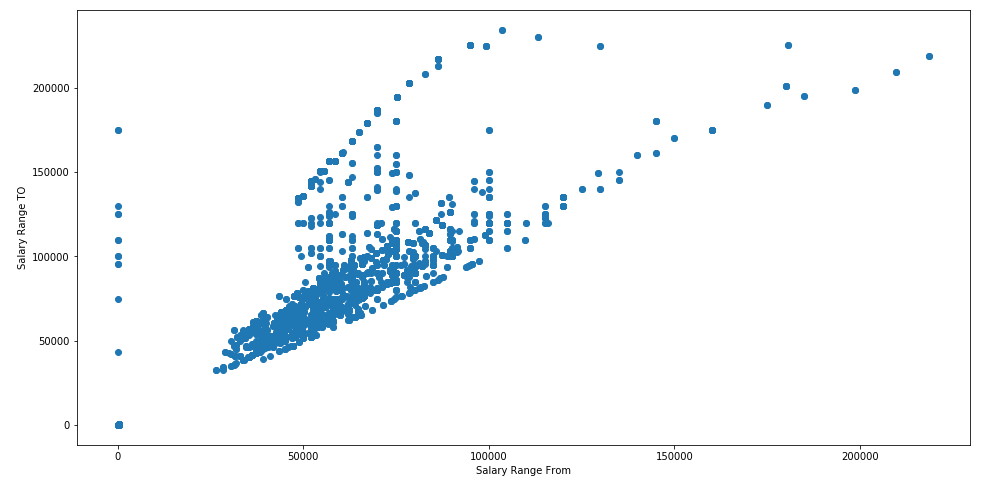### Visualization

Once we have changed the data according to the requirements, it is necessary to analyze this data. One such way of doing this is by visualizing the results. A better visual representation helps in an optimal analysis of the data projections.

The following is an example to visualize the data.:

###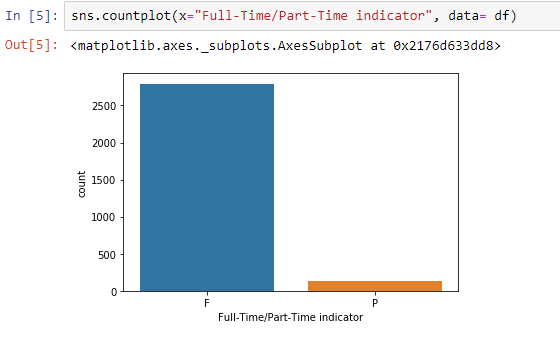Bar graph of full vs part-time workers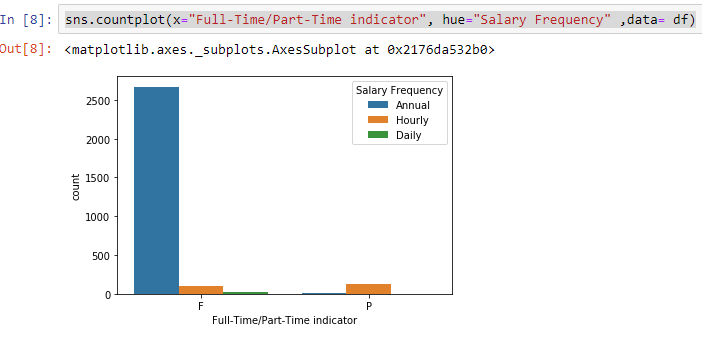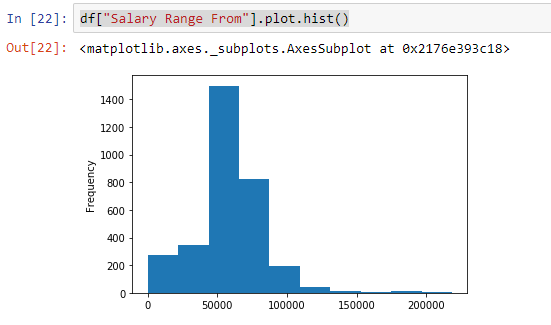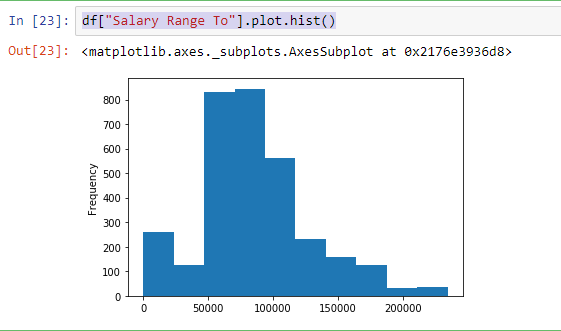``````import matplotlib.pyplot as plt
fig = plt.figure(figsize = (10,10))
ax = fig.gca()
sns.heatmap(df1.corr(), annot=True, fmt=".2f")
plt.title("Correlation",fontsize=5)
plt.show()``````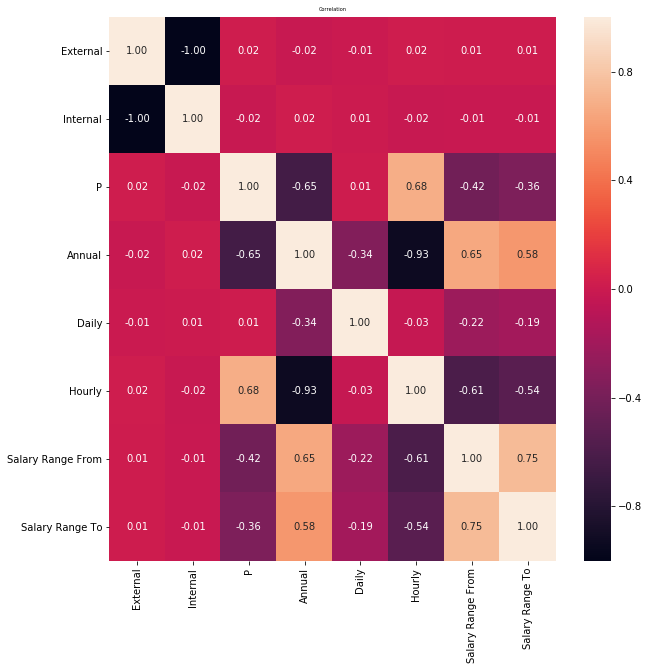### Analysis

After visualization, we can make our analysis looking at the various plots and graphs. Suppose we are working on job data, by looking at the visual representation of a particular job in a region we can make out the number of jobs in a particular domain.

From the above analysis, we can assume the following results

• The number of part-time jobs in the data set is very less compared to full-time jobs.
• while part-time jobs stand at less than 500, full-time jobs are more than 2500.
• Based on this analysis, We can build a prediction model.

Have any questions? mention them in the comments of this article on Anaconda Python, and we will get back to you as soon as possible.

Topics:
anaconda ,python

Comment (1)

Save
{{ articles.views | formatCount}} Views

Published at DZone with permission of Mohammad Waseem . See the original article here.

Opinions expressed by DZone contributors are their own.